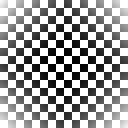# Cantor Tuples

Cantor pairs (and triplets, or tuples in general) are way to enumerate multi-dimensional spaces, similar to the Z curve. Unlike the Z curve, Cantor Tuples don’t preserve locality very well.

The idea is very simple: we trace a curve back and forth across the space until we’ve covered the whole thing. Here are the definitions we’ll be using below:

• `type Dimensions = Int`
• How many `Dimensions` to work in
• `type Range = Int`
• A bounded or unbounded `Range` of coordinates
• `range n = if n == 0 then [0..] else [0..n]`
• A way to enumerate a `Range`
• `type Point = [Int]`
• Storing lists of coordinates rather than tuples lets us use any number of `Dimensions`
• `type Curve = [Point]`
• A `Curve` is a line traced through the space
• `data Shape = Shape {sDim :: Dimensions, sRange :: Range, sPred :: Point -> Bool}`
• We define a `Shape` using a predicate, deciding whether a `Point` is in the `Shape` or not

## Axis-aligned `Curves`

The most obvious way to trace a `Curve` across a `Shape` is to start at `(0, 0)` (which for our purposes will be the top left of the images) and increase, say, the `x` coordinate to get `(1, 0)`, then `(2, 0)`, etc. until we exhaust the `Shape`’s `Range`, then jump to `(0, 1)` and do the same thing, and so on:

``````aaCurve :: Range -> Dimensions -> Curve
aaCurve r 1 = [[x]  | x <- range r]
aaCurve r n = [x:xs | x <- range r, xs <- aaCurve r (n - 1)]

aaShape :: Shape -> Curve
aaShape (Shape d r p) = filter p (aaCurve r d)``````

If we use this to trace a black-to-white gradient across a square image, we get this: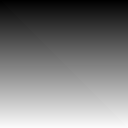### A Finite Example

Let’s say we have a `circle` (where `dim = 2 ^ scale =` `128` is the width and height of the following images):

``````pythagoras' :: Int -> Int -> Int
pythagoras' a b = a ^ 2 + b ^ 2  -- There's no need to take the square root

circle :: Shape
circle = let centre = dim `div` 2
p [x, y] = pythagoras' (x - centre) (y - centre) < (centre ^ 2)
in Shape 2 (2 * centre) p``````

Here’s what we get when we use an axis-aligned curve to trace a black-to-white gradient through the `circle`:

``````circlePixels = aaPixels circle

drawCircle x y = let this = DM.lookup [x, y] circlePixels
in maybe 255 id this``````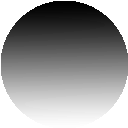Aside

Another thing we can do with `circle` is to approximate pi:

• `pi * r^2` is the area of any circle
• `r = dim `div` 2` in our example
• `sRange s ^ sDim s` is the area of the bounding box of `s :: Shape`
• `sRange circle = dim = 2 * r`
• `sDim circle = 2`
• `boxArea = (2 * r)^2 = 4 * r^2` for `circle`
• `circleArea / boxArea = (pi * r^2) / (4 * r^2) = pi / 4` follows from simple algebra
• Therefore `pi = 4 * circleArea / boxArea`
• Since each `Point` is an uniform distance from its neighbours, counting how many are in a `Shape` is a measure of the `Shape`’s area
• `aaArea = length . aaShape` gives us the area inside a `Shape`
• `circleArea = fromIntegral \$ aaArea circle`
• `boxArea = fromIntegral \$ aaArea (Shape (sDim circle) (sRange circle) (const True))`
• Plugging these values into our definition of pi gives `3.088516315125293`
• Increasing the radius decreases the error, since the sampling gives a less ‘jagged’ approximation of our circle

### An Unbounded Example

What happens if our `Range` is unbounded (ie. `range 0`)? For example, we might define an infinitely-repeating pattern:

``````checkerboard :: Shape
checkerboard = let f        = (`mod` 2) . (`div` 8)
g [x, y] = f x == f y
in Shape 2 0 g``````

Obviously we can’t draw the whole pattern, but we can draw a small section:

``drawBoard x y = sPred checkerboard [x, y]``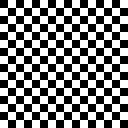Since the area of `checkerboard` is infinite, so is the length of a `Curve` traced through it. However, an infinite `Curve` returned by `aaCurve` will not contain every `Point` in the `Shape`.

This is because we only add a `Point` from the second row once we’ve reached the end of the first row; since the first row never ends, we’ll never add a `Point` from any other row!

## Diagonal Traces

Cantor’s approach handles infinite patterns like `checkerboard` by tracing diagonal lines across the shape, from one coordinate axis to another:

• Each `Point` on the `x` axis is the start of a diagonal line
• To get from one `Point` in a line to the next, we decrement the `x` coordinate and increment the `y`
• If we have more dimensions, we fix the first coordinate (ie. `x`) and recurse using the rest of the dimensions
• Once the `x` coordinate hits `0`, we jump to the start of the next line
``````cantorMax [_]    = True
cantorMax (x:xs) = sum xs == 0

cantorStart n 1 = [n]
cantorStart n d = 0 : cantorStart n (d-1)

cantorNext :: Point -> Point
cantorNext (x:xs) | cantorMax (x:xs) = xs ++ [1+x]
cantorNext (x:xs) | cantorMax xs     = (x + 1) : cantorStart (sum xs - 1) (length xs)
cantorNext (x:xs) | otherwise        = x : cantorNext xs

-- A full curve
cantorCurve :: Range -> Dimensions -> Curve
cantorCurve r d = takeWhile ((<= 2 * r) . sum) (iterate cantorNext (replicate d 0))

cantorShape :: Shape -> Curve
cantorShape (Shape d r p) = filter p (cantorCurve r d)``````

To see how this works, we can trace a black-to-white gradient across a square, following the curve given by `cantorCurve`. Compare it to the axis-aligned version above:We start in the top left corner and draw a diagonal line from the top edge to the left edge, then draw another next to it, and another next to it, and so on. Note that Cantor’s method naturally draws a triangle; to make it trace a square, we’ve had to filter out those points which extend too far to the right or the bottom.

### A Finite Example

Let’s revisit our `circle`. If we trace a gradient across it using Cantor’s method, we get the following: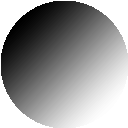### Unbounded Example

If we apply this to the checkerboard pattern, we’re now guaranteed to reach any finite coordinate in finite time: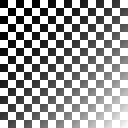### Removing All Bounds

The checkerboard example is unbounded on the right and bottom, but does have a bound on the top and the left. Cantor’s method can exploit this to zig-zag across the pattern, but it doesn’t rely on there being any bounds. Instead of zig-zagging, we can follow a spiral, starting from any point, and be guaranteed to eventually reach all points. For example, if we treat the checkerboard as unbounded in all directions: